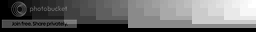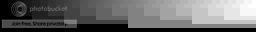## Something I found out about the Neo Geo colors

d3m1ck
Posts: 1
Joined: Tue May 07, 2019 4:48 am

### Something I found out about the Neo Geo colors

So, it is speculated that this is how the palette is generated,
and this via MAME:

220 O = RGB 4
470 O = RGB 3
1000 O = RGB 2
2200 O = RGB 1
3900 O = RGB 0
8200 O = DAK OUT

How they look:But really, what if what's below is true (5VDC reference):

.15625 V / 220 O = -.000710 A = RGB 4
.3125 V / 470 O = -.000664 A = RGB 3
.625 V / 1000 O = -.000625 A = RGB 2
1.25 V / 2200 O = -.000568 A = RGB 1
2.5 V / 3900 O = -.000641 A = RGB 0

Total RGB Amps * .95 = DAK OUT

7790 O total resistance) / 8200 O = 95% of current

Note: Regardless of the voltage going into the chip itself (I just use 5V as a reference seeing as I understand most boards are powered by 5VDC anyway), it seems that voltage - whatever it may be - is divvied up this way to represent the binary system required for palette generation, and the resistances are used to even everything out for channel level generation for color, the DAK resistance to dim the overall RGB color information as a whole (at 95% the total), just as described in official documents.

If that's the case, then MAME forgot Ohm's Law! Ohm's Law, for those unfamiliar, is the relationship between Voltage, Current, and Resistance. As per Ohm's Law, resistance R is proportional to current I, but voltage V is inversely proportional to both resistance R and current I.

To further understanding, see these equations:

V = I * R
I = V / R
R = V / I

Ohm's law is a triangular relationship, as it were:

V
-----
I | R

To be fair, though, MAME IS on to something although they just used resistor values instead of derived current values. Below are my derived 32 values in milliamps based on 5V (should hold true for any voltage, though):

0
710
1374
2084
2709
3419
4083
4793
5361
6071
6735
7445
8070
8780
9444
10154
10795
11505
12169
12879
13504
14214
14878
15588
16156
16866
17530
18240
18865
19575
20239
20949

so really:

1 = 000710 (3%) cRGB 8 d8
2 = 001374 (7%) cRGB 18 d17
4 = 002709 (13%) cRGB 33 d31
8 = 005361 (26%) cRGB 67 d64
16 = 010795 (51%) cRGB 130 d124
DAK = 95% * [R, G, B] cALL

These are the levels I derived:From all of this, I was able to determine that .020949 Amps (per 5VDC reference) is the total current with all channel bits set (MAX R G B, or white) and that any current under it divided by the max generates a percent, which can then be used to derive 24 bit values, like how I have them above. Label "d" denotes DAK set.

I remember viewing the Sengoku Denshou High Score screen and seeing a level clash somewhere near the middle of the gradient on it. NOW I feel I have derived the palette correctly, and that MAME's method is as of yet incomplete, though they pretty much nailed everything else.

The way the DAK bit works is evident on the top most portion of the above image. It would seem that the DAK bit is far from perfect (how SNK/ADK implemented it), but can still be used anyway with some finagling.

Current edit: To be even clearer, despite the actual voltage provided for the colors, the percentages should still remain the same, and even if the Amps derived are of smaller values, they should still behave like the Ohms Law derived ones at the beginning of this post.

Return to “MAME Discussion”

### Who is online

Users browsing this forum: No registered users and 2 guests